Question

# Please show work :) Complete the following using ordinary interest. (Use Days in a year table.)...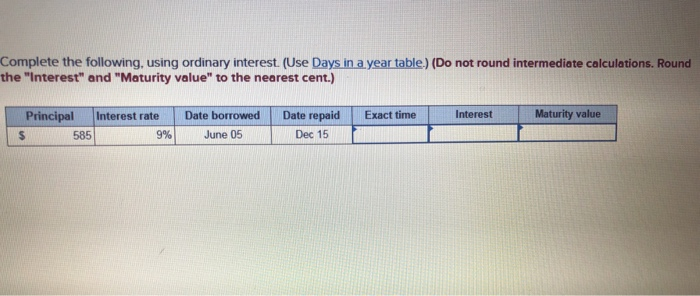Complete the following using ordinary interest. (Use Days in a year table.) (Do not round intermediate calculations. Round the "Interest" and "Maturity value to the nearest cent.) Exact time Interest Maturity value Principal Interest rate \$ 585 9% Date borrowed June 05 Date repaid Dec 15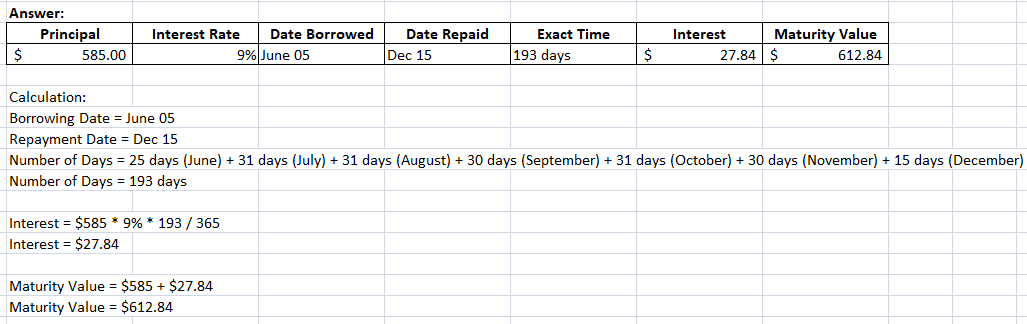#### Earn Coins

Coins can be redeemed for fabulous gifts.

Similar Homework Help Questions
• ### Complete the following using exact interest. (Use Days in a year table) (Do not round intermediate...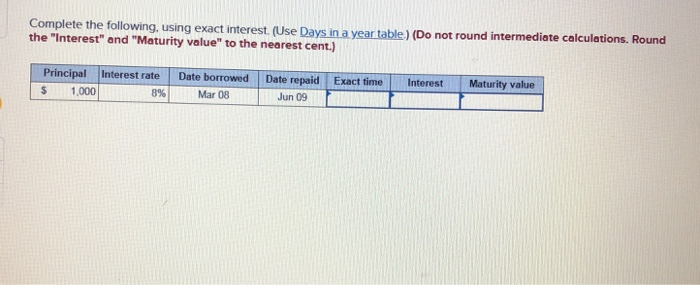Complete the following using exact interest. (Use Days in a year table) (Do not round intermediate calculations. Round the "Interest" and "Maturity value to the nearest cent.) Interest rate Exact time Interest Maturity value Principal \$ 1.000 Date borrowed Mar 08 Date repaid Jun 09

• ### Complete the following using exact interest. (Use Days in a vear table.) (Do not round intermediate...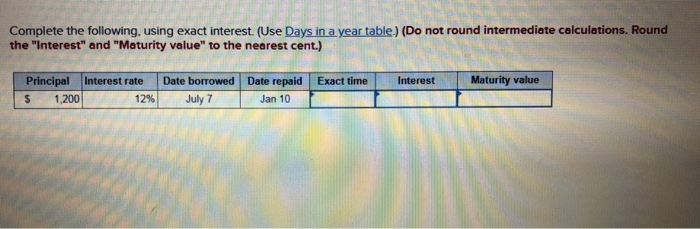Complete the following using exact interest. (Use Days in a vear table.) (Do not round intermediate calculations. Round the "Interest" and "Maturity value to the nearest cent.) Exact time Interest Maturity value Principal \$ 1,200 Interest rate 12% Date borrowed Date repaid July 7 Jan 10

• ### Use ordinary interest: (Round your answer to the nearest cent.) Interest Date Date Principal Rate Borrowed...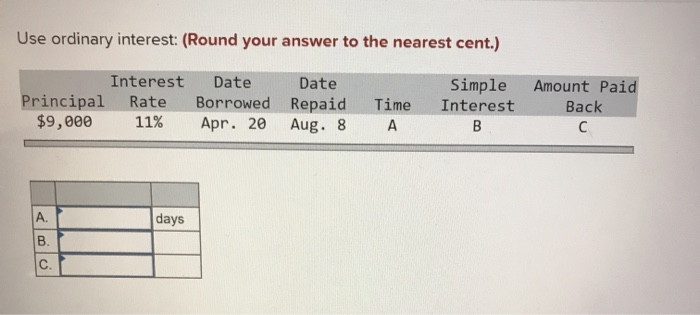Use ordinary interest: (Round your answer to the nearest cent.) Interest Date Date Principal Rate Borrowed Repaid \$9,000 11% Apr. 20 Aug. 8 A Time Simple Interest B Amount Paid Back days

• ### Given Principal \$14,5ee, Interest Rate 8%, Time 24e days (use ordinary interest) Partial payments: On 108th...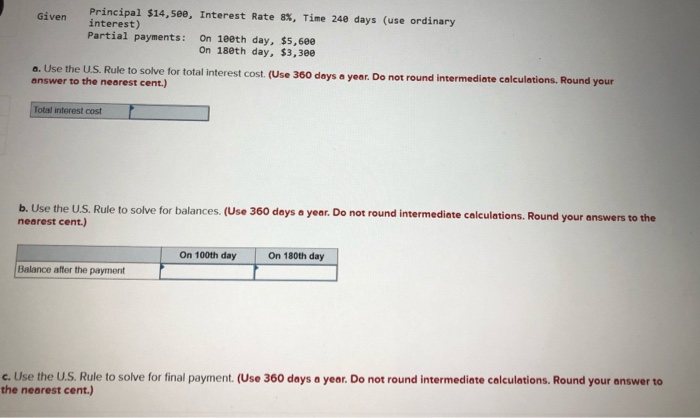Given Principal \$14,5ee, Interest Rate 8%, Time 24e days (use ordinary interest) Partial payments: On 108th day, \$5,6ee On 18eth day, \$3,300 6. Use the U.S. Rule to solve for total interest cost. (Use 360 days a year. Do not round Intermediate calculations. Round your answer to the nearest cent.) Total interest cost | b. Use the U.S. Rule to solve for balances. (Use 360 days a year. Do not round intermediate calculations. Round your answers to the nearest cent.)...

• ### Complete the following for the present value of an ordinary annuity (Please use the following provided...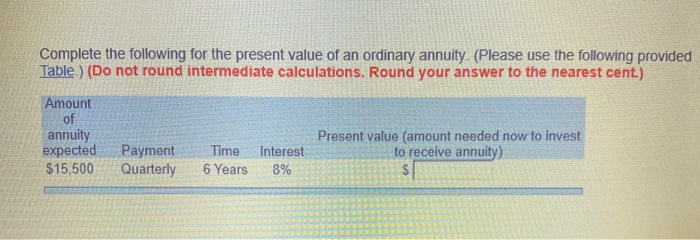Complete the following for the present value of an ordinary annuity (Please use the following provided Table ) (Do not round intermediate calculations. Round your answer to the nearest cent.) Amount annuity expected \$15,500 Present value (amount needed now to invest to receive annuity) Payment Quarterly Time 6 Years Interest 8%

• ### Complete the ordinary annuity. (Please use the following provided Table.) (Do not round intermediate calculations. Round...

Complete the ordinary annuity. (Please use the following provided Table.) (Do not round intermediate calculations. Round your answer to the nearest cent.) Amount of payment \$11 700 Payment payable- semiannually.Years 9 Interest rate 6% Value of annuity ?

• ### Determine the maturity date and compute interest for each note. (Use 360 days a year. Do...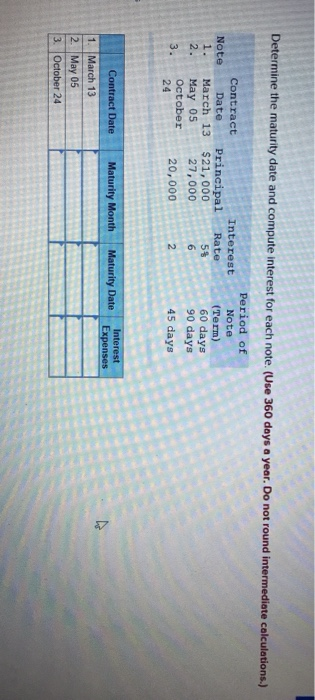Determine the maturity date and compute interest for each note. (Use 360 days a year. Do not round intermediate calculations.) Note 1. Interest Rate Contract Date Principal March 13 \$21,000 May 05 27,000 October 20,000 5\$ Period of Note (Term) 60 days 90 days 45 days 24 Maturity Month Maturity Date Interest Expenses Contract Date 1. March 13 2. May 05 3. October 24

• ### Determine the maturity date and compute interest for each note. (Use 360 days a year. Do...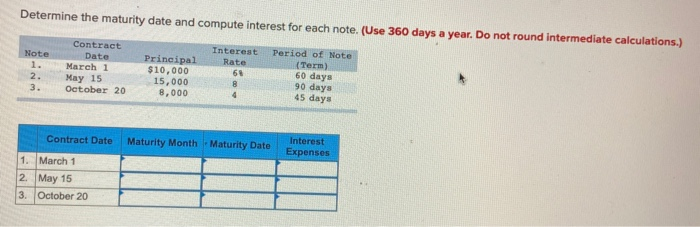Determine the maturity date and compute interest for each note. (Use 360 days a year. Do not round intermediate calculations.) Note Contract Date March 1 May 15 October 20 Principal \$10,000 15,000 8,000 Interest Period of Note Rate (Term 60 days 90 days 45 days Contract Date Maturity Month - Maturity Date Interest Expenses 1. March 1 2. May 15 3. October 20

• ### Shawn Bixby borrowed \$26,000 on a 150-day, 9% note. After 70 days, Shawn paid \$2,900 on the note. On day 107, Shawn paid an additional \$4,900. Use ordinary interest.

Shawn Bixby borrowed \$26,000 on a 150-day, 9% note. After 70 days, Shawn paid \$2,900 on the note. On day 107, Shawn paid an additional \$4,900. Use ordinary interest. a. Determine the total interest use the U.S. Rule. (Do not round intermediate calculations. Round your answer to the nearest cent.)     Total interest             \$      b. Determine the ending balance due use the U.S. Rule. (Do not round intermediate calculations. Round your answer to the nearest cent.)     Ending balance due            \$

• ### Complete the following table for the simple discount notes. Use the ordinary interest method. (Use 360...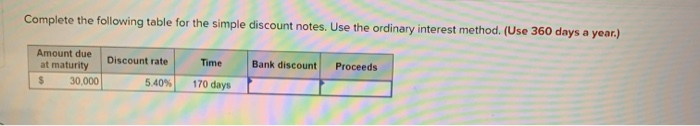Complete the following table for the simple discount notes. Use the ordinary interest method. (Use 360 days a year.) Bank discount Proceeds Amount due at maturity 30.000 Discount rate 5.40% Time 170 days